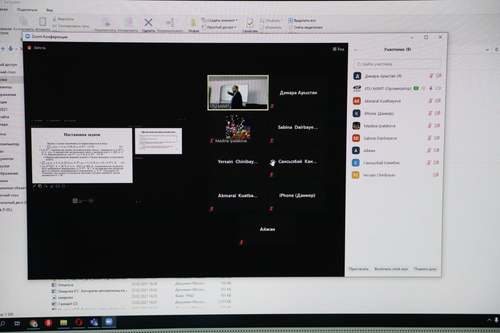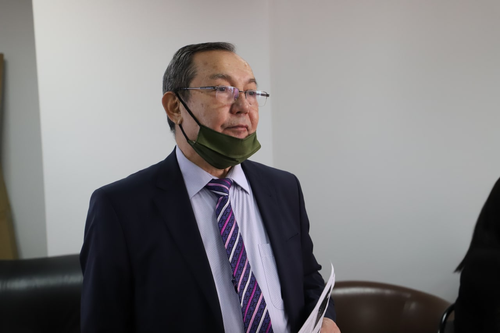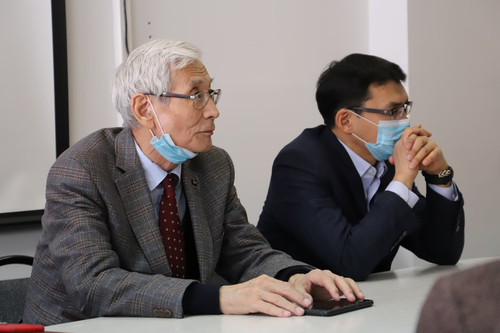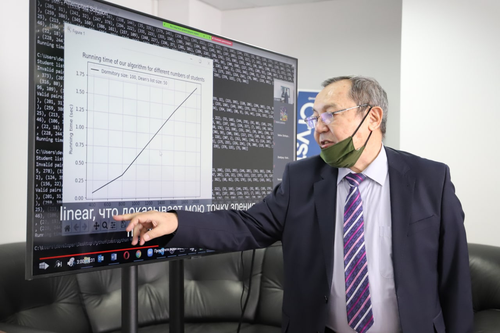IITU
P=NP?
26.02.2021

The question of the equality of the complexity classes P and NP has been one of the central open problems in the theory of algorithms for more than three decades. The relations between the classes P and NP are discussed in a section of algorithm theory called computational complexity theory.

The problem of equality of classes P and NP is one of the seven problems of the millennium, for the solution of which the Clay Mathematical Institute has awarded a prize of one million US dollars. Our teacher, Doctor of Technical Sciences of the Department "Information Systems", Professor Sinchev Baktygerey Kuspanovich presented his version of the solution to this problem at a scientific seminar.

In his report "P vs. NP or on the polynomial solvability of NP-complete", Baktygerey Kuspanovich noted the problems of solving this problem. The P=NP problem is as follows: if a positive answer to a question can be quickly checked (in polynomial time), then is it true that the answer to this question can be quickly found (in polynomial time and using polynomial memory).

Baktygerey Kosanovich invited research seminar in Dubai EXPO-2021. We wish good luck to our professor with the solution of a difficult problem!Версия сайта для слабовидящих